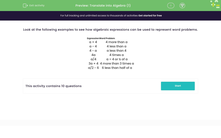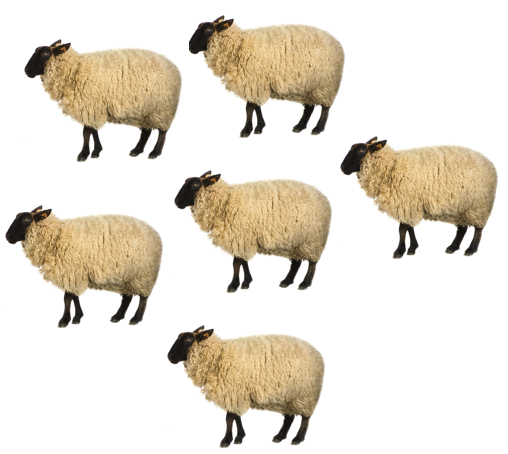# Translate Word Problems into Algebra

In this worksheet, students will choose the expression that matches the given word problem.Key stage:  KS 3

Curriculum topic:   Algebra

Curriculum subtopic:   Use and Interpret Algebraic Notation

Popular topics:   Algebra worksheets, Year 7 Algebra worksheets

Difficulty level:#### Worksheet Overview

Look at the following examples to see how algebraic expressions can be used to represent word problems.

Example 1

Katie eats a cookies and her brother Freddie eats 5 more than Katie.

We can write this as an algebraic expression:

Freddie eats a + 5 cookies.Example 2

There are x number of sheep in a field and y number of cows.

The farmer doubles the number of sheep.

How many animals are there in the field now? Write this as an algebraic expression.

There are now 2x + y animals in the field.In the questions that follow, we're going to practise using algebra to express word problems.

Here is a list of some of the most important ways to express word problems using algebra. Have a read through and then we'll start on the questions.

Expression Word Problem
a + 4 4 more than a
a - 4 4 less than a
4 - a a less than 4
4a 4 times a
a/4  a ÷ 4 or ¼ of a
3a + 4  4 more than 3 times a
a/2 - 6  6 less than half of a

### What is EdPlace?

We're your National Curriculum aligned online education content provider helping each child succeed in English, maths and science from year 1 to GCSE. With an EdPlace account you’ll be able to track and measure progress, helping each child achieve their best. We build confidence and attainment by personalising each child’s learning at a level that suits them.

Get started